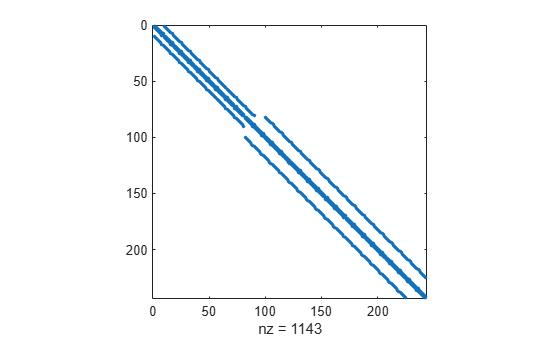nnz

Number of nonzero matrix elements

Description

example

N = nnz(X) returns the number of nonzero elements in matrix X.

Examples

collapse all

Create an identity matrix and determine the number of nonzeros it contains.

X = eye(4)
X = 4×4

1     0     0     0
0     1     0     0
0     0     1     0
0     0     0     1

N = nnz(X)
N = 4

Use nnz in conjunction with a relational operator to determine how many matrix elements meet a condition. Since relational operators produce logical matrices of 1s and 0s, the nnz function counts the 1s where the condition is true.

Create a matrix and determine how many elements are greater than 10.

X = magic(5)
X = 5×5

17    24     1     8    15
23     5     7    14    16
4     6    13    20    22
10    12    19    21     3
11    18    25     2     9

nnz(X>10)
ans = 15

The density of a matrix is the ratio of nonzeros to the total number of elements, nnz(X)/numel(X).

Create a sparse matrix representing the finite difference Laplacian on an L-shaped domain and calculate its density.

X = delsq(numgrid('L',20));
spy(X)d = nnz(X)/numel(X)
d = 0.0194

The result indicates that only about 2% of the elements in the matrix are nonzero.

Input Arguments

collapse all

Input matrix.

Data Types: single | double | int8 | int16 | int32 | int64 | uint8 | uint16 | uint32 | uint64 | logical | duration | calendarDuration
Complex Number Support: Yes